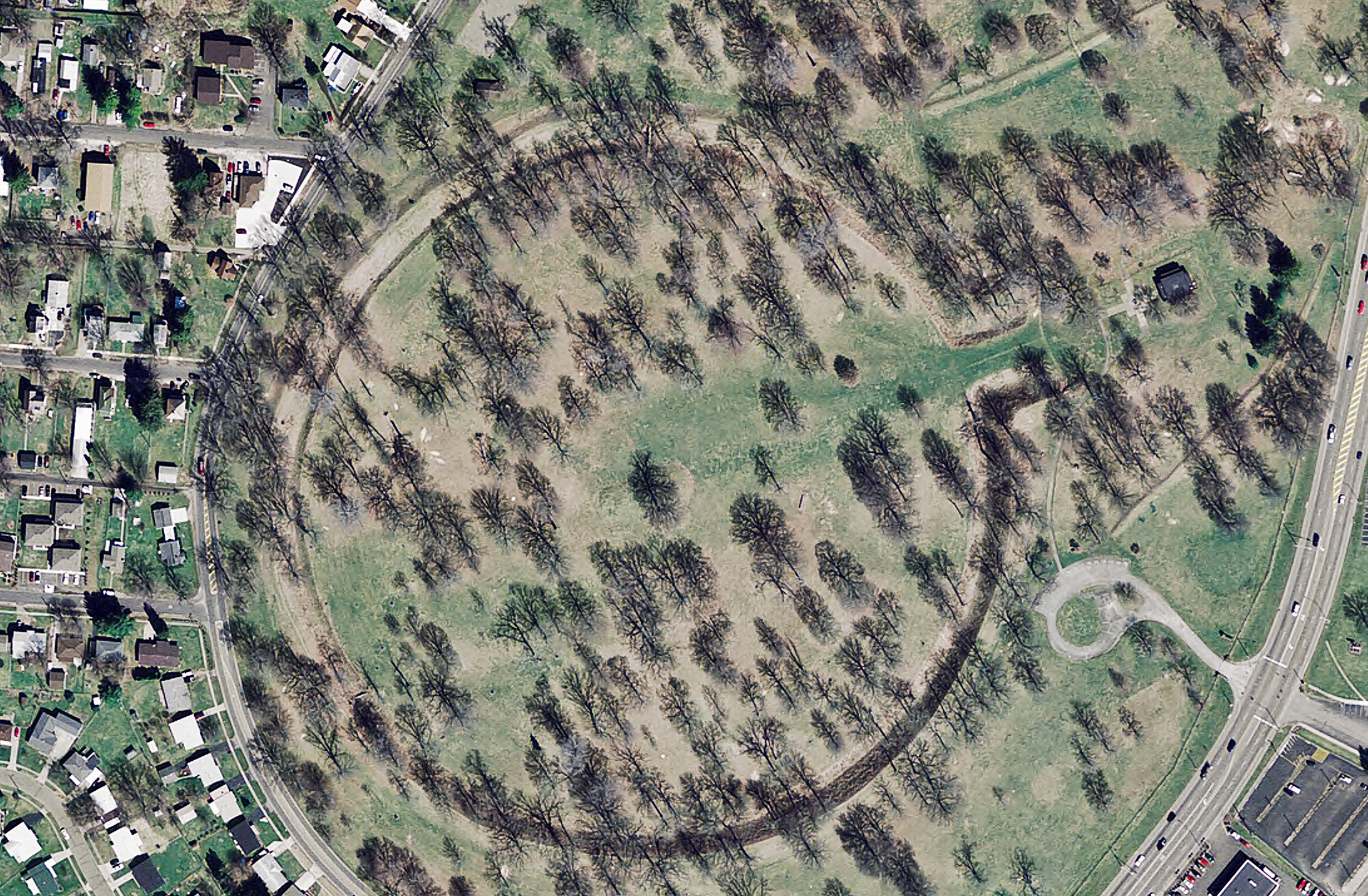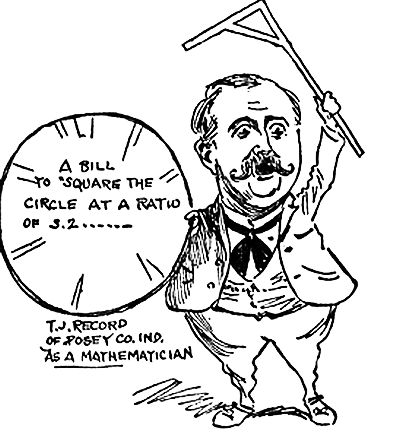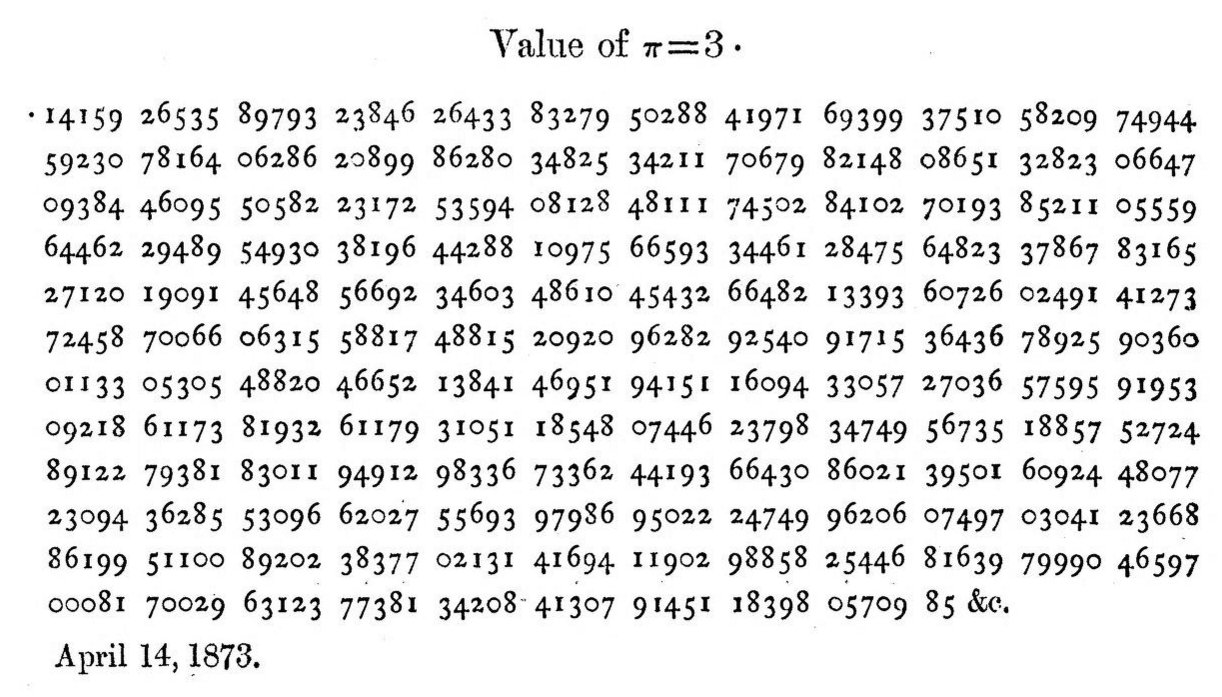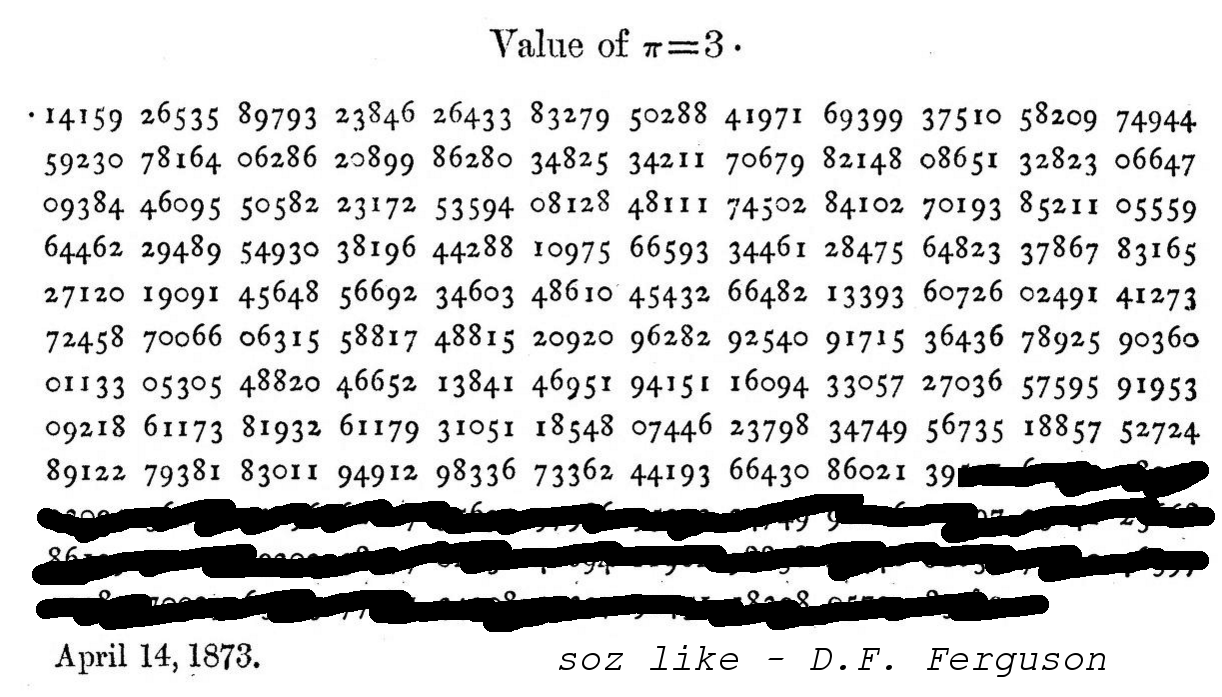π = 3.14159265358979323846264338327950288419716939937510582097494459230781640628620899862803482534211706798214808651328230664709384460955058223172535940812848111745028410270193852110555964462294895493038196442881097566593344612847564823378678316527120190914564856692346034861045432664821339360726024914127372458700660631...

## What do we know about π?

• It has to do with circles - something something 2πr
• It tastes delicious
• It's infinite
• It goes on forever
• It has infinitely many decimal digits
• It's random
• There's no pattern to its digits
• It's irrational
• It's transcendental

# How to calculate π

## Measure a circle## Measure a pendulum's swing

$\pi = \frac{T}{2} \sqrt{\frac{L}{g}}$

## Embrace the noise

$x^2 + y^2 \leq 1$

π ≈ 4 × 0 / 0?

### Draw a polygon which looks like a circle

$P_{2n} = \frac{2p_nP_n}{p_n+P_n}, \\[1.5em] p_{2n} = \sqrt{p_nP_{2n}}$

## Give up and legislate it

"the ratio of the diameter and circumference is as five-fourths to four."## Machin's formula

$\frac{\pi}{4} = 4 \arctan \left(\frac{1}{5}\right) - \arctan \left(\frac{1}{239}\right)$

Rewrite as an infinite series

$\pi = 4 \sum_{n=0}^{\infty} \frac{(-1)^n)}{2n+1} \left( 4 \left(\frac{1}{5}\right)^{2n+1} - \left(\frac{1}{239}\right)^{2n+1} \right)$

## William Shanks, Local Hero

William Shanks spent over 20 years calculating π to 707 decimal places.## William Shanks, Local Hero

William Shanks spent over 20 years calculating π to 707 decimal places.

But he made an error at the 528th digit.## William Shanks, Local Hero

William Shanks spent over 20 years calculating π to 707 decimal places.

But he made an error at the 528th digit.## Use a machine

D.F. Ferguson spotted Shanks's error and broke his record with a mechanical desk calculator.

Since then, computers have gone on to compute a stupid number of decimal places of π.# Spigot algorithms

(A bit like this bear, but for digits of π)

## Spigot algorithms

Iterative algorithms which produce digits one at a time, and never reuse a digit in a later step.

And they only use a constant amount of working memory.So I bought

three.onefouronefivenine.com

## The Bailey-Borwein-Plouffe formula

$\pi = \sum_{k=0}^{\infty} \frac{1}{16^k}\left( \frac{4}{8k+1} - \frac{2}{8k+4} - \frac{1}{8k+5} - \frac{1}{8k+6} \right)$

You can extract any digit of π without working out any of the previous ones.